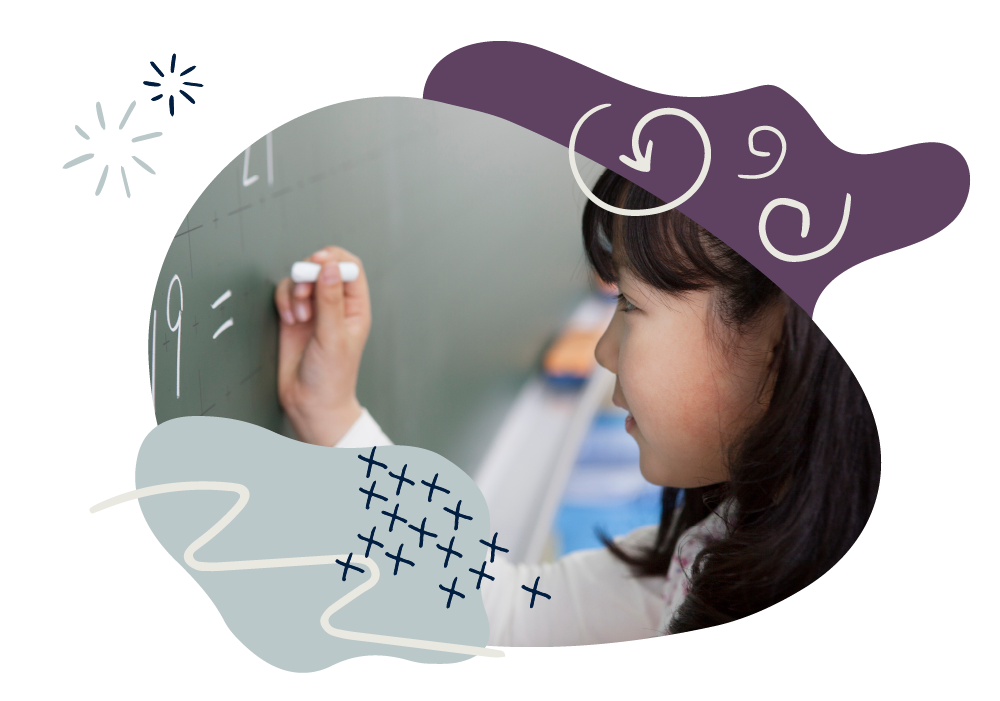We use cookies to enhance your experience on our website. By continuing to use our website, you are agreeing to our use of cookies. You can change your cookie settings at any time. Find out more# Maths: Age 9–10 (Year 5)

In Year 5, your child will continue to practise mental and written calculation methods and use all the operations to solve problems.

They will meet prime, square and cube numbers, and work with factors and multiples. They will calculate perimeters and areas, draw and measure angles, convert between units of time and learn some imperial measures. They will reflect and translate shapes on coordinate grids and interpret line graphs and timetables.

They’ll be taught long multiplication and multiply four-digit by two-digit numbers, then short division for dividing four-digit by one-digit numbers. They’ll begin to multiply fractions, learn more about decimals and be introduced to percentages. They will be able to count in powers of 10 and round numbers up to 1,000,000 to the nearest 10, 100, 1000, 10,000 and 100,000.

Don’t worry if some methods that your child learns are new to you! There are still simple things you can do at home to support their development.

## How to help at home

You don’t need to be an expert to support your child with maths or help them develop a good sense of number! Here are three simple but effective learning ideas that you can try with your child at home.

### 1. Dice with decimals

Make ‘×’ ‘÷’ and ‘10’, ‘100’, ‘1000’ cards to place face down in two piles. Roll a dice four times to create a number (e.g. 4258), then insert a decimal point somewhere (e.g. 42.58). Take a card from each pile and do the calculation (e.g. 42.58 ÷ 100 = 0.4258).

### 2. Play Battleship games

Play Battleships by drawing ships on coordinate grids. Try to sink each other’s ships by guessing their positions using coordinates, such as (1,2). Remember that the first number in the coordinate bracket is on the horizontal x-axis. The second number is on the vertical y-axis.

### 3. Hit the sales

Sales in shops, catalogues or online are great for working with percentages. For example, in a 20% off sale, if the full price (that is 100%) of an item is £10, how much is the item discounted by (£2) and what will the sale price be (£8)?

You’ll find more activity ideas in our Kids’ activities section

## What your child will learn at school

#### Number & place value in Year 5 (age 9–10)

In Year 5, children will be expected to read, write, order and compare numbers up to 1,000,000, recognising the place value of each digit. This includes:

• counting forwards and backwards with positive and negative numbers
• rounding numbers up to one million to the nearest 10, 100, 1000, 10 000 and 100 000
• recognising Roman numerals I, V, X, L, C, D and M to read numbers and years.

#### Addition & subtraction in Year 5 (age 9–10)

In Year 5, children will be expected to be able to solve addition and subtraction problems involving numbers with more than four digits. This includes:

• practising a range of mental calculation strategies as well as using a variety of methods to calculate including using objects, diagrams or drawings such as number lines, models such as the area/grid method and written column addition and column subtraction
• using rounding to estimate answers and checking that their answers are sensible and accurate
• solving multi-step word problems for which they will need to solve multiple calculations before coming to the final answer.

#### Multiplication & division in Year 5 (age 9–10)

In Year 5, children will be expected to be able to solve multiplication and division problems involving numbers up to four digits and begin to learn long multiplication. This includes:

• multiplying four-digit numbers by two-digit numbers
• dividing four-digit numbers by one-digit numbers and interpreting remainders
• understanding the terms multiple, factor, common factor, prime, square and cube numbers.

#### Fractions, decimals & percentages in Year 5 (age 9–10)

In Year 5, children will be expected to be able to find fractions of numbers and quantities including thousandths and begin to learn about percentages. This includes:

• recognising and converting between mixed numbers and improper fractions
• multiplying proper fractions and mixed numbers by whole numbers
• understanding percentages as ‘the number of parts per hundred’ and knowing percentage and decimal equivalents of  and .

#### Geometry in Year 5 (age 9–10)

In Year 5, children will be expected to be able to solve problems about shapes by reasoning using their knowledge of geometric properties. This includes:

• drawing and measuring angles in degrees
• finding missing angles at a point, on a line and in rectangles
• describing translations and reflections of shapes on a four-quadrant coordinate grid.

#### Measurement in Year 5 (age 9–10)

In Year 5, children will be expected to be able to convert between metric units of measurement and solve problems involving decimal measurements, money and time. This includes:

• calculating the perimeter and area of shapes (including those made up of squares and rectangles)
• estimating volumes of 3D shapes and capacities of containers
• learning some imperial units, such as inches, pounds and pints.